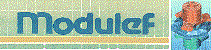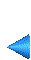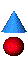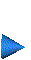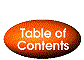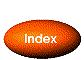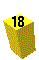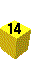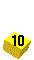## 2.6.2 Keywords

POIN : Definition of the characteristic points .

• POIN  (A) : the keyword to activate
• IMPRE (I) : print parameter for execution
• NPOINT (I) : number of points to describe
• Loop from J=1 to NPOINT, the number of characteristic points
• NOP (I) : the point number
• NOREF (I) : its reference
• X (R) : the abscissa
• Y (R) : the ordinate
• Z (R) : the height
• end of loop J

Remarks:

• The points may be entered in any order (due to NOP).
• If the points do not lend itself to a manual capturing (due to their number or position), but are easier to capture via a subroutine, we can use subroutine SPDPTS, which is called by module APNOP3. This is done by:

• fixing the value of NPOINT to -NPOINT, and omitting the loop,
• supplying subroutine SPDPTS as follows:

SUBROUTINE SPDPTS(NPOINT,MPPOI,MNRPC,XY)

where

NPOINT
is the number of points (input),
MPPOI(*)
is the integer array linking local point I to global point J=MPPOI(I),
MNRPC(*)
is the array of point references (indexed by I), and
XY(3,*)
is the real array of point coordinates (indexed by I), i.e.,
X = XY(1,.), Y = XY(2,.), Z = XY(3,.).

INTR : Calls module SDREST to introduce  a mesh residing on file into main memory in order to modify it.

• INTR  (A) : the keyword to activate
• IMPRE (I) : print parameter for execution
• NINOPO (I) : the level of the D.S. to introduce into m.m.
• NOM (A) : the name of the file containing the D.S.

Remark:

• To be usable by the geometric modification modules, the meshes introduced in this way must be of type P1, i.e., the nodes are the vertices.

MA23 : Calls module MA2D3E to create a 3D mesh from the data of a 2D mesh of one of the sections of the domain. The latter is thus topologically a cylinder defined by a stacking   constructed from the given section.

• MA23  (A) : the keyword to activate
• IMPRE (I) : print parameter for execution
• NINOPE (I) : the level of the I.D.S. serving as data
• NINOPS (I) : the level of the resulting O.D.S.

The data belonging to MA23XX, the preprocessor which corresponds to module MA2D3D, then follows, to which we will return to later (chapter 3).

SU23 : Calls module TN2D3D to create a surface  from the data of a 2D mesh.

• SU23  (A) : the keyword to activate
• IMPRE (I) : print parameter for execution
• NINOPE (I) : the level of the I.D.S. serving as data
• NINOPS (I) : the level of the resulting O.D.S.
• FONINT (I) : option to give the projection function defining the map from the 2D mesh to that of the surface:
• 0 : the projection function F2D3D is given in the form of a subroutine (see chapter 4, module TN2D3D);
• 1 : the function is input via a function parser, i.e. the data must be input in the following form (see chapter 1, keyword COUR):

• 'X01(X,Y) = function of X and Y ;'
the expression must be between ' and terminated with a ;
• 'Y01(X,Y) = function of X and Y ;'
• 'Z01(X,Y) = function of X and Y ;'
• FIN(A) : indicates the end of data for this function

Remark:

• X01, Y01 and Z01 defines the coordinates (x,y and z) of the surface points from those (X and Y) of the initial mesh points.

MANU : Calls the mesh generator CONOPO which corresponds to a manual description , i.e., from points (keyword POIN).

• MANU  (A) : the keyword to activate
• IMPRE (I) : print parameter for execution
• LEVEL (I) : the level of the resulting D.S.
• NE (I) : the number of elements to create
• Loop from J=1 to NE, the number of elements
• NCGE (I) : type of element: 1point, 2segment, 3triangle,
4quadrangle, 5tetrahedra, 6pentahedra, 7hexahedra;
• NUDSD (I) : the sub-domain number of the element
• Loop from K=1 to the number of element vertices
.
NUMSOM(K) (I) : the number of element vertices

• end of loop K
• Loop from L=1 to the number of element edges (if NTYP > 2)
.
NREFA(L) (I) : the reference number of the element edges

• end of loop L
• Loop from M=1 to the number of element faces (if NTYP > 4)
.
NREFF(M) (I) : the reference number of the element faces

• end of loop J

Remarks:

• NUMSOM(*) is one of the points created with keyword POIN.
• The order of the vertices, edges and faces is the standard order of the D.S. NOPO.

OBJE : Calls the mesh generator CONOPO corresponding to the case of a single element.

• OBJE  (A) : the keyword to activate
• IMPRE (I) : print parameter for execution
• LEVEL (I) : the level of the resulting D.S.
• NCGE (I) : the type of element: 1point, 2segment, 3triangle,
4quadrilateral, 5tetrahedron, 6pentahedron, 7hexahedron;

• IREF (I) : reference option
• 0 : all references are set to 0
• 1 : the references are different:
1. for the vertices: 1, 2, 3, 4, ..., n
2. for the edges: n+1, ..., n+p
3. for the faces: n+p+1, ...,

Remarks:

• The sub-domain number is set to 1.
• The keyword NUME can be used to modify the numbers.
• This module is used when doing simple tests rapidly.

TRAN : Calls module MODNOP to generate a mesh resulting from a translation  of a given mesh.

• TRAN  (A) : the keyword to activate
• IMPRE (I) : print parameter for execution
• LEVEL1 (I) : level of the initial D.S.
• LEVEL2 (I) : level of the resulting D.S.
• NBNNF (I) : the number of reference numbers to change
• NBNNSD (I) : the number of sub-domain numbers to change
• Loop from J=1 to NBNNF (if NBNNF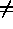0)
• NOLD(J) (I) : the reference number to change
• NNEW(J) (I) : the number to assign to items referenced by NOLD(J)
• end of loop J
• Loop from J=1 to NBNNSD (if NBNNSD0)
• NOLD(J) (I) : the sub-domain number to change
• NNEW(J) (I) : the number to assign to items referenced by NOLD(J)
• end of loop J
• TRANX (R) : the x component of the translation
• TRANY (R) : the y component of the translation
• TRANZ (R) : the z component of the translation

ROTA : Calls module MODNOP to generate a mesh resulting from a rotation  of a given mesh.

• ROTA  (A) : the keyword to activate
• IMPRE (I) : print parameter for execution
• LEVEL1 (I) : level of the initial D.S.
• LEVEL2 (I) : level of the resulting D.S.
• NBNNF (I) : the number of reference numbers to change
• NBNNSD (I) : the number of sub-domain numbers to change
• Loop from J=1 to NBNNF (if NBNNF0)
• NOLD(J) (I) : the reference number to change
• NNEW(J) (I) : the number to assign to items referenced by NOLD(J)
• end of loop J
• Loop from J=1 to NBNNSD (if NBNNSD0)
• NOLD(J) (I) : the sub-domain number to change
• NNEW(J) (I) : the number to assign to items referenced by NOLD(J)
• end of loop J
• VX (R) : the first component of the axis of rotation
• VY (R) : its second component
• VZ (R) : its third component
•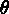(R) : the rotation angle in degrees
• XC (R) : the abscissa of the center of rotation
• YC (R) : the ordinate
• ZC (R) : the height

SYMP : Calls module MODNOP to generate the mesh resulting from symmetry  with respect to a plane in a given mesh.

• SYMP  (A) : the keyword to activate
• IMPRE (I) : print parameter for execution
• LEVEL1 (I) : level of the initial D.S.
• LEVEL2 (I) : level of the resulting D.S.
• NBNNF (I) : the number of reference numbers to change
• NBNNSD (I) : the number of sub-domain numbers to change
• Loop from J=1 to NBNNF (if NBNNF0)
• NOLD(J) (I) : the reference number to change
• NNEW(J) (I) : the number to assign to items referenced by NOLD(J)
• end of loop J
• Loop from J=1 to NBNNSD (if NBNNSD0)
• NOLD(J) (I) : the sub-domain number to change
• NNEW(J) (I) : the number to assign to items referenced by NOLD(J)
• end of loop J
• A (R) : the parameters defining the plane of symmetry given in the form
AX + BY + CZ + D = 0.
• B (R) : the second coefficient of the plane equation
• C (R) : the third coefficient
• D (R) : the fourth coefficient

Remark:

• SYMD is similar to SYMP.

DILA : Calls module MODNOP to generate the mesh resulting from a dilation  of given mesh.

• DILA  (A) : the keyword to activate
• IMPRE (I) : print parameter for execution
• LEVEL1 (I) : level of the initial D.S.
• LEVEL2 (I) : level of the resulting D.S.
• NBNNF (I) : the number of reference numbers to change
• NBNNSD (I) : the number of sub-domain numbers to change
• Loop from J=1 to NBNNF (if NBNNF0)
• NOLD(J) (I) : the reference number to change
• NNEW(J) (I) : the number to assign to items referenced by NOLD(J)
• end of loop J
• Loop from J=1 to NBNNSD (if NBNNSD0)
• NOLD(J) (I) : the sub-domain number to change
• NNEW(J) (I) : the number to assign to items referenced by NOLD(J)
• end of loop J
• DILX (R) : the dilation coefficient in the x direction
• DILY (R) : the dilation coefficient in the y direction
• DILZ (R) : the dilation coefficient in the z direction
• XINV (R) : the abscissa of a point which is invariant by this transformation
• YINV (R) : the ordinate
• ZINV (R) : the height

TETR : Calls module DTRI3D to split  each element of the given mesh into tetrahedra.

• TETR  (A) : the keyword to activate
• IMPRE (I) : print parameter for execution
• LEVEL1 (I) : level of the initial D.S.
• LEVEL2 (I) : level of the resulting D.S.

RETR : Calls module RETRIN to split  each element of a given mesh into sub-elements of the same type.

• RETR  (A) : the keyword to activate
• IMPRE (I) : print parameter for execution
• LEVEL1 (I) : level of the initial D.S.
• LEVEL2 (I) : level of the resulting D.S.
• N (I) : the splitting parameter

Remark:

• Each element is split into N� elements of the same type (N in the case of a segment, N� for triangles and quadrangles, or else N�).

NUME : Calls module MODNOP to modify attributes (reference or sub-domain numbers) in a given mesh.

• NUME  (A) : the keyword to activate
• IMPRE (I) : print parameter for execution
• LEVEL1 (I) : level of the initial D.S.
• LEVEL2 (I) : level of the resulting D.S.
• NBNNF (I) : the number of reference numbers to change
• NBNNSD (I) : the number of sub-domain numbers to change
• Loop from J=1 to NBNNF (if NBNNF0)
• NOLD(J) (I) : the reference number to change
• NNEW(J) (I) : the number to assign to items referenced by NOLD(J)
• end of loop J
• Loop from J=1 to NBNNSD (if NBNNSD0)
• NOLD(J) (I) : the sub-domain number to change
• NNEW(J) (I) : the number to assign to items referenced by NOLD(J)
• end of loop J

RECO : Calls module RECOLC to "glue together"  two meshes to form a new mesh.

• RECO  (A) : the keyword to activate
• IMPRE (I) : print parameter for execution
• LEVEL1 (I) : level of the first initial D.S.
• LEVEL2 (I) : level of the second initial D.S.
• LEVEL3 (I) : level of the resulting D.S.
• EPS (R) : the precision of the union (for example 0.01)
• IOPT (I) : the gluing option: 0 geometrical, 1 physical.
• NBNNF (I) : the number of reference numbers to change
• NBNNSD (I) : the number of sub-domain numbers to change
• Loop from J=1 to NBNNF (if NBNNF0)
• NOLD(J) (I) : the reference number to change
• NNEW(J) (I) : the number to assign to items referenced by NOLD(J)
• end of loop J
• Loop from J=1 to NBNNSD (if NBNNSD0)
• NOLD(J) (I) : the sub-domain number to change
• NNEW(J) (I) : the number to assign to items referenced by NOLD(J)
• end of loop J

Remarks:

• A geometrical "gluing together" consists of identifying the points by taking only their positions into account.
• On the contrary, a physical "gluing together" consists of identifying the points by taking their position and reference number into account (two points are said identical if their positions and references are the same).

ADPO : Calls module ADPNOP to define the nodes  of a given mesh (as opposed to a mesh for which the nodes are identical to the vertices).

• ADPO  (A) : the keyword to activate
• IMPRE (I) : print parameter for execution
• LEVEL1 (I) : level of the initial D.S.
• LEVEL2 (I) : level of the resulting D.S.
• N1 (I) : the number of nodes on the edges (endpoints excluded)
• ISET (I) : the number of internal nodes in each triangle or triangular face
• ISEQ (I) : the number of internal nodes in each quadrilateral or quadrilateral face
• ISETE (I) : the number of internal nodes in each tetrahedron
• ISEPE (I) : the number of internal nodes in each pentahedron
• ISEHE (I) : the number of internal nodes in each hexahedron
• NOESOM (I) :
1 : vertices are nodes
0 : if not

Remarks:

• It is no longer possible to activate the preceding keywords, consequently this keyword is called once the entire geometry has been processed.
• Renumbering is desired after this operation.

EXTR : Calls module TRCOAC to construct a D.S. NOPO containing the faces possessing a given reference number.

• EXTR  (A) : the keyword to activate
• IMPRE (I) : print parameter for execution
• LEVEL1 (I) : level of the initial D.S.
• LEVEL2 (I) : level of the resulting D.S.
• NBNNF (I) : the number of face reference numbers to consider
• Loop from J=1 to NBNNF (if NBNNF0)
• NREF(J) (I) : the face reference numbers to retain
• end of loop J

RENC : Calls module GIBBS to renumber  the nodes of a mesh.

• RENC  (A) : the keyword to activate
• IMPRE (I) : print parameter for execution
• LEVEL1 (I) : level of the initial D.S.
• LEVEL2 (I) : level of the resulting D.S.

Remark:

• This keyword usually comes just before saving the final mesh on file.

RENE : Calls module GIBBS to renumber  the elements and the nodes of a mesh.

• RENE  (A) : the keyword to activate
• IMPRE (I) : print parameter for execution
• LEVEL1 (I) : level of the initial D.S.
• LEVEL2 (I) : level of the resulting D.S.

Remark:

• This keyword usually comes just before saving the final mesh on file.

COUL : Calls module COULE1 to renumber  the elements by coloring.

• COUL  (A) : the keyword to activate
• IMPRE (I) : print parameter for execution
• LEVEL1 (I) : level of the initial D.S.
• LEVEL2 (I) : level of the resulting D.S.
• LVECT (I) : the maximum number of elements per color
• NLC (I) : the number of curved boundaries described
• NOMTAB (A) : the name of the array to be associated with the D.S.
• Loop from J=1 to NLC, the number of curved lines
• NREF(J) (I) the reference number of line J
• end of loop J

Remark:

• This keyword is used to regroup the elements of a mesh into disjoint sets. Consequently, it enables us to perform a calculation, for example of element matrices, on super-calculators.

SAUV : Calls module SAUVER to store  a mesh, residing in main memory, on file.

• SAUV  (A) : the keyword to activate
• IMPRE (I) : print parameter for execution
• NINOPO (I) : level of the D.S. to be saved on file
• NTNOPO (I) : the number of arrays to associate to the D.S. (usually 0)
• NOM (A) : the file name
• Loop from J=1 to NTNOPO (if NTNOPO0)
• NOMTAB (A) : the name of array J (4 characters)
• NTYP (I) : its type
• LONG (I) : the number of words
• Loop from K=1 to the number of variables
• VAL(K) (NTYP) : the variables of the array
• end of loop K
• COMMENT (A) : comment regarding the contents of array J
• end of loop J

Remark:

• This keyword may be activated at any time to save a partial or final mesh.

INTR : Calls module SDREST to introduce  a mesh residing on file into main memory.

• INTR  (A) : the keyword to activate
• IMPRE (I) : print parameter for execution
• NINOPO (I) : the level of the D.S. after it is introduced into m.m.
• NOM (A) : the name of the file containing the D.S.

Remark:

• In order to be usable by the geometrical transformation modules, a mesh introduced in this way must be of type P1, i.e., their nodes and vertices must coincide.

TUER : Calls module TUERSD to remove one or several mesh data structures (D.S.) from memory.

• TUER  (A) : the keyword to activate
• IMPRE (I) : print parameter for execution
• NBRE (I) : the number of D.S. to "kill"
• Loop from J=1 to NBRE
• LEVEL(J) (I) : the level of D.S. J to delete from the arrays
• end of loop J

Remark:

• This keyword can be activated to economize on memory space.

IMPR : Call module IMNOPO to print  the contents of a mesh data structure (D.S.).

• IMPR  (A) : the keyword to activate
• IMPRE (I) : print parameter for execution
• NINOPO (I) : the level of the D.S. to print

DESS : Calls module TRNOPO to plot  a mesh.

• DESS  (A) : the keyword to activate
• ITERM1 (I) : the terminal number in the nomenclature of your installation of the software package F3D
• ITERM2 (I) : set 0
• IOPT (I) : the mesh plotting option: 1 the edges, 2 the visible edges, 3 the invisible edges, 4 the shrunken mesh, 5 the same, visible, 6 the same, invisible, 7 all the elements, 8 the same, shrunken.
• LEVEL (I) : the level of the D.S. to plot

Remarks:

• The couple (ITERM1, ITERM2) defines the graphics device.
• The preprocessor TRNOXX is more frequently used to plot meshes.
• Consequently, by deleting this keyword from the APNOP3 facilities, we avoid loading the graphics libraries at the time the meshes are conceived.

FIN : Request to terminate the job.

• FIN  (A) : the keyword to activate

In addition, the following keywords are found in preprocessor APN3XX:

LIRE
: request to read  another data file (of the same format).
CONT
: request to suppress the data coherence controls .